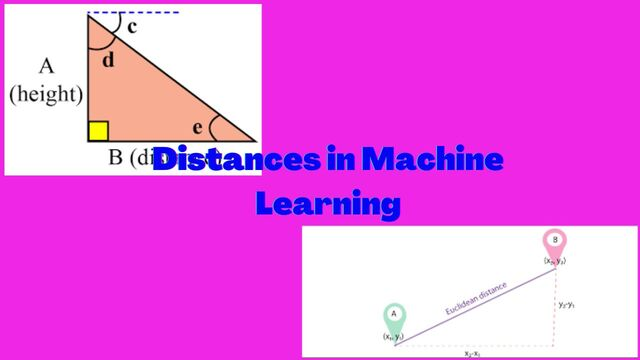# Distances in Machine Learning# Distances in Machine Learning

Every sample, record, word, sentence, object, image etc in the Machine learning language is called vector. If we want to measure the similarity or dissimilarity between two data points then we need distance function.

The choice of distance function depends on the specific task at hand and the properties of the data. For example,

• the Euclidean distance is often used for tasks such as classification and regression,
• the Jaccard distance is often used for tasks such as text classification,
• the Mahalanobis distance is often used for tasks such as fraud detection.
• the Manhattan distance is often used for tasks such as clustering.

## Euclidean distance:

This is the most common distance metric. It measures the distance between two points in a Euclidean space.

## Manhattan distance:

This distance metric measures the distance between two points in a city block. It is also known as the L1 norm.

## Minkowski distance:

This is a generalization of the Euclidean and Manhattan distances. It is defined by a parameter p, and the value of p determines the type of distance metric. For example, p=1 is the Manhattan distance, p=2 is the Euclidean distance, and p=infinity is the Chebyshev distance.

## Hamming distance:

This distance metric measures the distance between two binary vectors. It is defined as the number of bits that are different between the two vectors.

## Cosine similarity:

This distance metric measures the similarity between two vectors. It is defined as the cosine of the angle between the two vectors.

## Jaccard distance:

This distance metric measures the similarity between two sets. It is defined as the size of the intersection of the two sets divided by the size of the union of the two sets.

## Overlap coefficient:

This distance metric is similar to the Jaccard distance, but it is normalized to the range [0, 1].

## Sokal-Michener distance:

This distance metric measures the similarity between two biological sequences. It is defined as the number of shared matches divided by the total number of characters in the two sequences.

## Mahalanobis distance:

This distance metric is a generalization of the Euclidean distance. It takes into account the covariance of the data points.

## Wasserstein distance:

This distance metric measures the difference between two probability distributions. It is often used in machine learning tasks such as anomaly detection and clustering.

## Chebyshev distance:

This distance metric measures the maximum difference between two points. It is defined as the maximum absolute difference between the corresponding coordinates of the two points.

## Canberra distance

This distance metric is a variation of the Manhattan distance. It is defined as the sum of the absolute differences between the corresponding coordinates of the two points, divided by the sum of the absolute values of the coordinates.

## Kendall tau distance:

This distance metric measures the difference between the rankings of two items. It is defined as the sum of the number of times the two items are ranked in the opposite order.

## Spearman’s rank correlation coefficient:

This distance metric measures the correlation between the rankings of two items. It is defined as the Pearson correlation coefficient between the ranks of the two items.

## Jensen-Shannon divergence:

This distance metric measures the difference between two probability distributions. It is defined as the average of the Kullback-Leibler divergences between the two distributions.

Tags:

Updated: